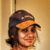# Create Linear transformation tool for raster using ArcPy

1031
4
11-24-2020 07:44 AM
Labels (2)by
New Contributor III

I am trying to convert a raster file with values ranging from 3.2 to 8.8 into a linear scale from 0 to 1. The conditions that i want to apply in the transformation are as follows: 3 to 5 gets 0, 5 to 7 Linear variation from o to 1 and gets values based on straight line equation y = (slope)x + c, And greater than 7 gets 1. This is an example and this may vary with others preferences.

So i want to create  a tool using Python code values in zero range(Ex:My case 3 to 5),  Linearly varying values from o to 1 range(Ex:My case 5 to 7),values in 1 range(Ex:My case greater than 7).How is it possible to create linear transformation as a tool in ArcGIS using python code where i can input those conditions mentioned above as parameters in the tool? I would like to send this tool to other users and so their conditions may vary based on it. What changes to be made in this code to make it work with the conditions.  I am a beginner and am relatively new to Python, so please excuse me if I sound a bit dumb 🙂

`import arcpyimport osimport sysfrom arcpy import envfrom arcpy.sa import *arcpy.CheckOutExtension("Spatial")env.workspace = r"H:\Test\Test1"arcpy.env.cellSize = 100Input = arcpy.GetParameterAsText(0)folder = arcpy.GetParameterAsText(1)raster = arcpy.GetParameterAsText(2)arcpy.AddMessage("Folder: {}".format(folder))arcpy.AddMessage("Raster: {}".format(raster))save_raster = os.path.join(folder, raster)arcpy.AddMessage("Save Location: {}".format(save_raster))output = RescaleByFunction("Elevation", TfLinear(Minimum, Maximum, "#", "#", "#", "#"), 0, 1)output.save(save_raster)`

Thank you:)

Tags (4)
4 RepliesbyMVP Frequent Contributor

Is there a rationale behind making a tool from an existing tool (and perhaps limiting its functionality)?by
New Contributor III

Hi David,

Thanks a lot  for the reply. The aim is to make the same tool a bit modified and in line with the terminologies that we use in the assignment. Example, i would like to write it as controlpoint1 = 3, controlpoint2 = 5, controlpoint3 = 7 and controlpoint4 = 8.8 for the example and would like to have just the linear trasformation. How could i add the control points in the code to create a simpler version of the same tool in ArcMap?

`import osimport sysfrom arcpy import envfrom arcpy.sa import *arcpy.CheckOutExtension("Spatial")env.workspace = r"H:\Test"arcpy.env.cellSize = 100inRaster = "elevation"Input = arcpy.GetParameterAsText(0)folder = arcpy.GetParameterAsText(1)raster = arcpy.GetParameterAsText(2)ControlPoint1 = arcpy.GetParameterAsText(3)ControlPoint2 = arcpy.GetParameterAsText(4)ControlPoint3 = arcpy.GetParameterAsText(5)ControlPoint4 = arcpy.GetParameterAsText(6)arcpy.AddMessage("Folder: {}".format(folder))arcpy.AddMessage("Raster: {}".format(raster))save_raster = os.path.join(folder, raster)arcpy.AddMessage("Save Location: {}".format(save_raster))minimum = ControlPoint2maximum = ControlPoint3lowerthresh = ControlPoint1valbelowthresh = 0upperthresh = ControlPoint4valabovethresh = 1myTfFunction = TfLinear(minimum, maximum, lowerthresh, valbelowthresh, upperthresh, valabovethresh)fromscale = 0toscale = 1outRescale = RescaleByFunction(inRaster, myTfFunction, fromscale, toscale)output.save(save_raster)`byMVP Frequent Contributor

without delving into the rescale function I'd say it looks ok. Maybe pass the controlpoints as floats:

e.g.

`ControlPoint1 = float(arcpy.GetParameterAsText(3))`

or just arcpy.GetParameter()

I'm no expert programmer, but I'd stop renaming variables for no reason, e.g. just call

`minimum = arcpy.GetParameterAsText(4)`

and so on.  It seems needlessly confusing otherwise.

Is there a specific part it's falling over on?  Do you know how to assign the parameters in the script tool itself?by
New Contributor III

Yes the tool has worked. Thank you so much 🙂